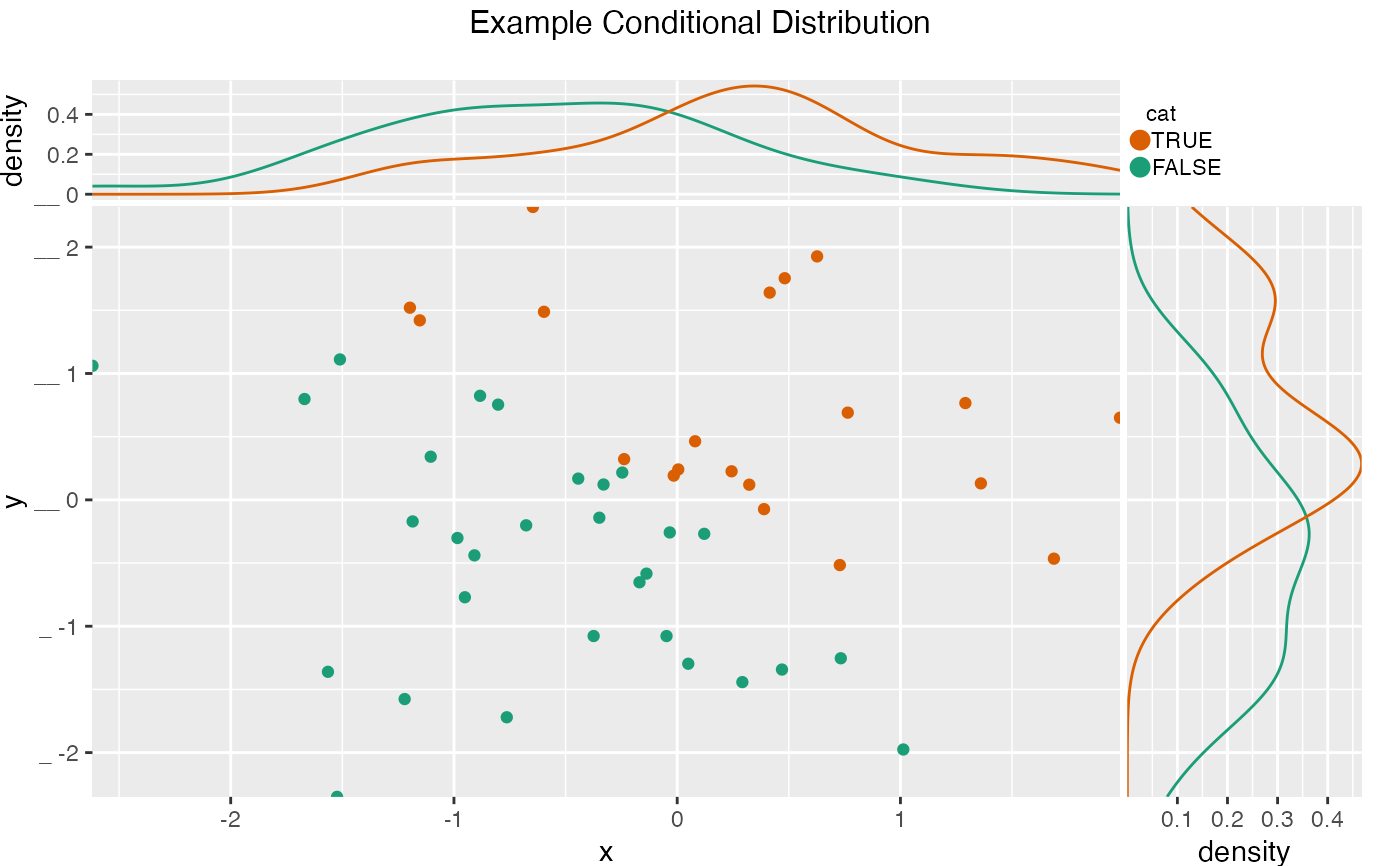Plot a scatter plot conditioned on a discrete variable, with marginal conditional density plots.

ScatterHistC(
frame,
xvar,
yvar,
cvar,
title,
...,
annot_size = 3,
colorPalette = "Dark2",
)

## Arguments

frame data frame to get values from name of the x variable name of the y variable name of condition variable title to place on plot no unnamed argument, added to force named binding of later arguments. numeric scale annotation text (if present) name of a Brewer palette (see https://colorbrewer2.org/ ) numeric: adjust x density plot numeric: adjust y density plot

plot grid

## Details

xvar and yvar are the coordinates of the points, and cvar is the discrete conditioning variable that indicates which category each point (x,y) belongs to.

ScatterHist

frm$cat <- frm$x+frm\$y>0